(Difference between revisions)
 Revision as of 19:19, 5 July 2019 (view source)Tohline (Talk | contribs) (→Two-Dimensional Configurations (Axisymmetric))← Older edit Revision as of 18:05, 3 August 2019 (view source)Tohline (Talk | contribs) (→Axisymmetric Equilibrium Structures)Newer edit → Line 327: Line 327: {| class="wikitable" style="margin-right: auto; margin-left: 75px; border-style: solid; border-width: 3px; border-color: black;" {| class="wikitable" style="margin-right: auto; margin-left: 75px; border-style: solid; border-width: 3px; border-color: black;" |- |- - ! style="height: 150px; width: 150px; background-color:lightgreen; border-right:2px solid black;" |[[User:Tohline/AxisymmetricConfigurations/SolutionStrategies#Axisymmetric_Configurations_.28Structure_.E2.80.94_Part_II.29|Constructing
Axisymmetric
Equilibrium
Configurations
]] + ! style="height: 150px; width: 150px; background-color:lightgreen; border-right:2px solid black;" |[[User:Tohline/AxisymmetricConfigurations/Equilibria|Constructing
Axisymmetric
Configurations
]] | | ! style="height: 150px; width: 150px; border-right:2px dashed black;" |[[User:Tohline/2DStructure/AxisymmetricInstabilities|Axisymmetric
Instabilities
to Avoid]] ! style="height: 150px; width: 150px; border-right:2px dashed black;" |[[User:Tohline/2DStructure/AxisymmetricInstabilities|Axisymmetric
Instabilities
to Avoid]]

Contents

 |   Tiled Menu   |   Tables of Content   |  Banner Video   |  Tohline Home Page   |

Spherically Symmetric Configurations

(Initially) Spherically Symmetric Configurations

Equilibrium Structures

1D STRUCTURE

Hydrostatic
Balance
Equation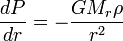$~\frac{dP}{dr} = - \frac{GM_r \rho}{r^2}$
Solution
Strategies

Isothermal
Sphere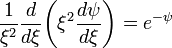$~\frac{1}{\xi^2} \frac{d}{d\xi}\biggl( \xi^2 \frac{d\psi}{d\xi} \biggr) = e^{-\psi}$
via
Direct
Numerical
Integration

Isolated
Polytropes
Lane
(1870)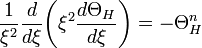$~\frac{1}{\xi^2} \frac{d}{d\xi}\biggl( \xi^2 \frac{d\Theta_H}{d\xi} \biggr) = - \Theta_H^n$
Known
Analytic
Solutions
via
Direct
Numerical
Integration
via
Self-Consistent
Field (SCF)
Technique

Free Energy
of
Bipolytropes

(nc, ne) = (5, 1)

Stability Analysis

1D STABILITY

Pressure-Truncated
Isothermal
Spheres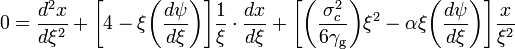$~0 = \frac{d^2x}{d\xi^2} + \biggl[4 - \xi \biggl( \frac{d\psi}{d\xi} \biggr) \biggr] \frac{1}{\xi} \cdot \frac{dx}{d\xi} + \biggl[ \biggl( \frac{\sigma_c^2}{6\gamma_\mathrm{g}}\biggr)\xi^2 - \alpha \xi \biggl( \frac{d\psi}{d\xi} \biggr) \biggr] \frac{x}{\xi^2}$ where: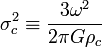$~\sigma_c^2 \equiv \frac{3\omega^2}{2\pi G\rho_c}$     and,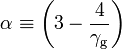$~\alpha \equiv \biggl(3 - \frac{4}{\gamma_\mathrm{g}}\biggr)$
via
Direct
Numerical
Integration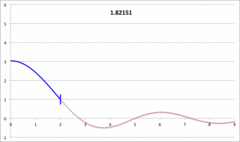Yabushita's
Analytic Sol'n
for
Marginally Unstable
Configurations
(1974)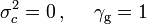$~\sigma_c^2 = 0 \, , ~~~~\gamma_\mathrm{g} = 1$ and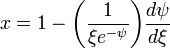$~x = 1 - \biggl( \frac{1}{\xi e^{-\psi}}\biggr) \frac{d\psi}{d\xi}$

Polytropes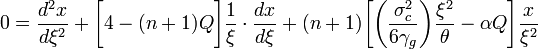$~0 = \frac{d^2x}{d\xi^2} + \biggl[ 4 - (n+1) Q \biggr] \frac{1}{\xi} \cdot \frac{dx}{d\xi} + (n+1) \biggl[ \biggl( \frac{\sigma_c^2}{6\gamma_g } \biggr) \frac{\xi^2}{\theta} - \alpha Q\biggr] \frac{x}{\xi^2}$ where: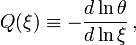$~Q(\xi) \equiv - \frac{d\ln\theta}{d\ln\xi} \, ,$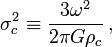$~\sigma_c^2 \equiv \frac{3\omega^2}{2\pi G\rho_c} \, ,$     and,$~\alpha \equiv \biggl(3 - \frac{4}{\gamma_\mathrm{g}}\biggr)$
Isolated
n = 3
Polytrope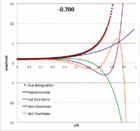Pressure-Truncated
n = 5
Configurations

Our Analytic Sol'n
for
Marginally Unstable
Configurations
(2017)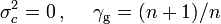$~\sigma_c^2 = 0 \, , ~~~~\gamma_\mathrm{g} = (n+1)/n$ and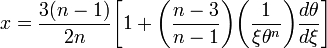$~x = \frac{3(n-1)}{2n}\biggl[1 + \biggl(\frac{n-3}{n-1}\biggr) \biggl( \frac{1}{\xi \theta^{n}}\biggr) \frac{d\theta}{d\xi}\biggr]$

1D DYNAMICS

Two-Dimensional Configurations (Axisymmetric)

(Initially) Axisymmetric Configurations

Axisymmetric Equilibrium Structures

2D STRUCTURE

Toroidal & Toroidal-Like

Definition: anchor ring

2D STABILITY

2D DYNAMICS

Two-Dimensional Configurations (Nonaxisymmetric Disks)

Infinitesimally Thin, Nonaxisymmetric Disks

2D STRUCTURE

Three-Dimensional Configurations

(Initially) Three-Dimensional Configurations

Equilibrium Structures

3D STRUCTURE
 "One interesting aspect of our models … is the pulsation characteristic of the final central triaxial figure … our interest in the pulsations stems from a general concern about the equilibrium structure of self-gravitating, triaxial objects. In the past, attempts to construct hydrostatic models of any equilibrium, triaxial structure having both a high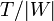$~T/|W|$ value and a compressible equation of state have met with very limited success … they have been thwarted by a lack of understanding of how to represent complex internal motions in a physically realistic way… We suggest … that a natural attribute of [such] configurations may be pulsation and that, as a result, a search for simple circulation hydrostatic analogs of such systems may prove to a fruitless endeavor. — Drawn from §IVa of Williams & Tohline (1988), ApJ, 334, 449
 Special numerical techniques must be developed "to build three-dimensional compressible equilibrium models with complicated flows." To date … "techniques have only been developed to build compressible equilibrium models of nonaxisymmetric configurations for a few systems with simplified rotational profiles, e.g., rigidly rotating systems (Hachisu & Eriguchi 1984; Hachisu 1986), irrotational systems (Uryū & Eriguchi 1998), and configurations that are stationary in the inertial frame (Uryū & Eriguchi 1996)." — Drawn from §1 of Ou (2006), ApJ, 639, 549

Binary Systems

• S. Chandrasekhar (1933), MNRAS, 93, 539: The equilibrium of distorted polytropes. IV. the rotational and the tidal distortions as functions of the density distribution
• S. Chandrasekhar (1963), ApJ, 138, 1182: The Equilibrium and the Stability of the Roche Ellipsoids
 Roche's problem is concerned with the equilibrium and the stability of rotating homogeneous masses which are, further, distorted by the constant tidal action of an attendant rigid spherical mass.

3D STABILITY

3D DYNAMICS